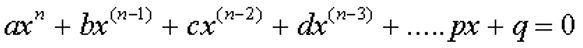# Learn Algebraic Number#What do you mean by Algebraic Number in math?

An online algebraic number definition

Algebraic numbers are the real or complex number solutions to polynomial equations of the form.The coefficients a, b, c, d, ....p, q, are integers or fractions. All rational numbers are algebraic while some irrational numbers are algebraic.#Related Number Types

##Math number calculation ►

Online Algebra calculation, formulas , Digital calculation, Statistical calculation, Math Converters Pet Age Calculator